# Convert Numbers to Roman Numerals

By Stephen Armstrong // March 24th, 2020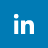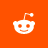## Learn how to add a Roman numeral converter to your game.

While they've totally fallen out of use in everyday life, Roman numerals still find their use in video games (such as Final Fantasy VII), movies (such as Rocky IV), and other multimedia set during the Roman period.

This tutorial will help you create a way to convert numbers into Roman numerals.

As this converter uses pure C# code, it can be used in any game engine or framework that uses C# (such as MonoGame and Unity).

## Create a RomanNumerals Class

Right click on your project in Solution Explorer and select > .In the Add New Item window, select Class and enter a Name. In this example we call it RomanNumerals.cs. Click Add when finished.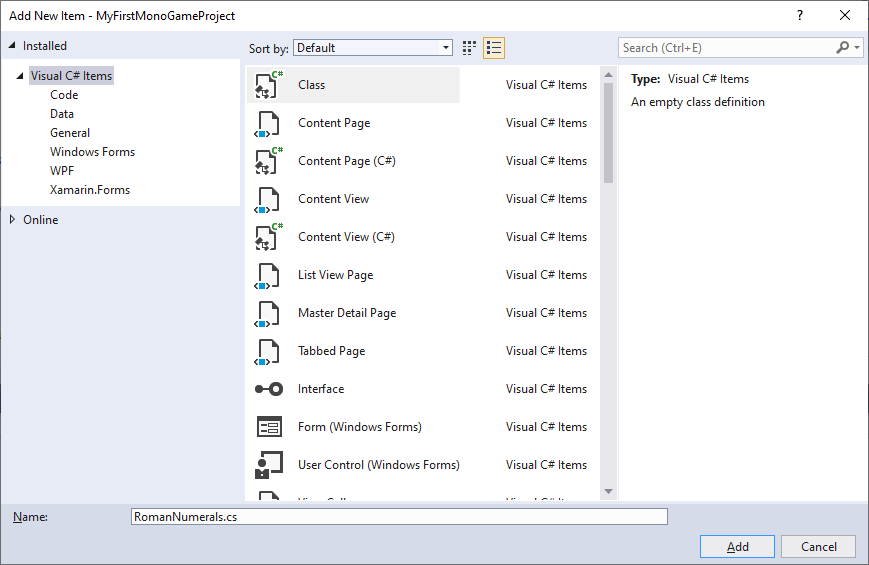RomanNumerals.cs should automatically open, and you'll see the following: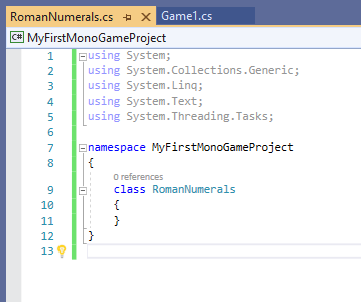Feel free to delete this default code, and replace it with the following:

• using System;
• class RomanNumerals
• {
• public static string ReturnRomanNumerals(ushort value)
• {
• }
• }

This is the basic frame of our converter, now it's time to add to it!

The first thing to do is to create three string arrays as fields. Add the following code to RomanNumerals as follows:

• // List the total potential Roman numerals, sorted into fields of hundreds, tens, and ones.
• static string[] hundreds = new string[] { "", "C", "CC", "CCC", "CD", "D", "DC", "DCC", "DCCC", "CM" };
• static string[] tens = new string[] { "", "X", "XX", "XXX", "XL", "L", "LX", "LXX", "LXXX", "XC" };
• static string[] ones = new string[] { "", "I", "II", "III", "IV", "V", "VI", "VII", "VIII", "IX" };

These arrays contain all the potential values for numbers between 1 - 999.

## Building the Converter

Go to public static string ReturnRomanNumerals(ushort value) and add the following:

The first thing you should probably do is add a handler for zero (0).

There is no 0 in Roman numerals, so you can either return a standard "0", or the Latin word "nulla" (meaning "none").

• // Immediately return a value for 0.
• if (value == 0) { return "nulla"; }

Next create a string to hold the Roman numerals that will be returned.

• // This string holds the value to be returned.
• string returnValue = "";

As there is only one symbol for thousands ("M"), a simple For loop will add sufficient "M"s to the returnValue.

• // Thousands: For every thousand, add an "M".
• for (int i = 0; i < value / 1000; i++)
• {
• returnValue += "M";
• }

Now we move onto hundreds and tens.

As we created the string array fields earlier, we need to get indexes for these arrays.

We can do so by dividing the value by 100 and 10 (for hundreds and tens respectively) and then by using the remainder of the resulting values divided by ten.

• // Hundreds
• //Create an index for the above "hundreds" field by dividing the value by 100.
• int hundredsIndex = (value / 100);
• // Set hundredsIndex as the remainder of hundredsIndex divided by 10.
• hundredsIndex %= 10;
• // Add the value from hundreds using hundredsIndex.
• returnValue += hundreds[hundredsIndex];
• // Tens
• // Create an index for the above "tens" field by dividing the value by 10.
• int tensIndex = (value / 10);
• // Set tensIndex as the remainder of tensIndex divided by 10.
• tensIndex %= 10;
• // Add the value from tens using the tensIndex.
• returnValue += tens[tensIndex];

It's similar for ones, only we just need to use the remainder of the value divided by 10.

• // Ones
• //Create an index for the above "ones" field by getting the remainder of the value divided by 10.
• int onesIndex = (value % 10);
• // Add the value from ones using the onesIndex.
• returnValue += ones[onesIndex];

The last thing to do is to return the returnValue.

• // Return the finished string.
• return returnValue;

## Testing

As a public static class, the Roman numeral converter can be called from anywhere.

For the easiest way to test it, go to Game1.cs and add the following:

• // Change this number to the number to convert to Roman numerals.
• ushort numberToChange = 1234;
• System.Console.WriteLine("{0} in Roman: {1}", numberToChange, RomanNumerals.ReturnRomanNumerals(numberToChange));

If you run the code now, check the Output and you should see the following: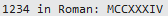Feel free to change numberToChange to whatever number you want - why not try the year you were born? For me, that would be 1984: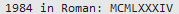For large-scale testing, you can use the Random Number Generator we created earlier, for example:

• // This 10-length array will store numbers as they are generated.
• ushort[] randomExamples = new ushort;
• // Loop through the array.
• for (byte i = 0; i < randomExamples.Length; i++)
• {
• // Generate a random number between 0 and 4000.
• randomExamples[i] = (ushort)RandomNumber.RandomInt(0, 4000);
• // Output the number.
• System.Console.WriteLine("Random number between 0 and 4000: {0}", randomExamples[i]);
• // Output the Roman numerals.
• System.Console.WriteLine("That number converted to Roman numerals: {0}", RomanNumerals.ReturnRomanNumerals(randomExamples[i]));
• }

Run this code, and you will see the following: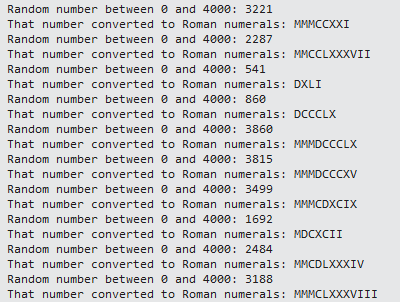As standard Roman numerals don't have anything higher than 1000, try not to pass numbers over 4000 to the converter.

< Go backWelcome to Industrian.net!

On this website you'll find more information about our games, and also some tutorials for you to start making games of your own! You can also follow us on various social platforms!Popular PostsLatest Posts